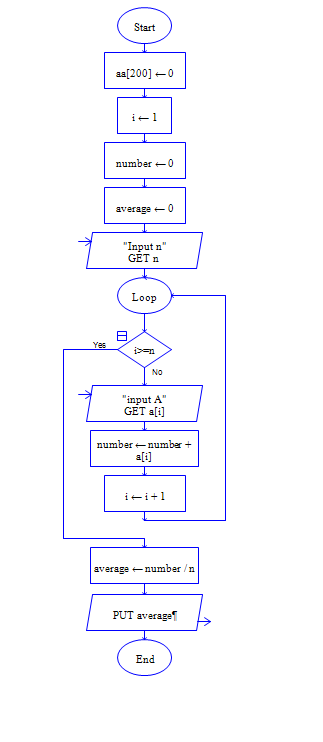# Average of list in python | without using built-in function

Python Program to get list size n and n elements of list, then compute sum and average of the elements.

Sample Input 1:

5 5 7 9 3 1

Sample Output 1:

25 5.0

#### Flow Chart Design#### Program or Solution

``` l=list(map(int,input("Enter array elements:").split(" "))) sum1=0 for i in l: sum1+=i print(sum1/len(l)) ```

#### Program Explanation

Input: To get list of numbers seprated by space, use split(" ") method.

Split() method splits the numbers as seprate elements.

By default this methods are considered as string, since input() method returs string.

Use map() function to convert all elements as integer and store it in list.

Process: for statement takes the elements in the list l one by one as value of i, and adds i on each iteration with sum1.

Finally sum1/len(l) is average of list elements.